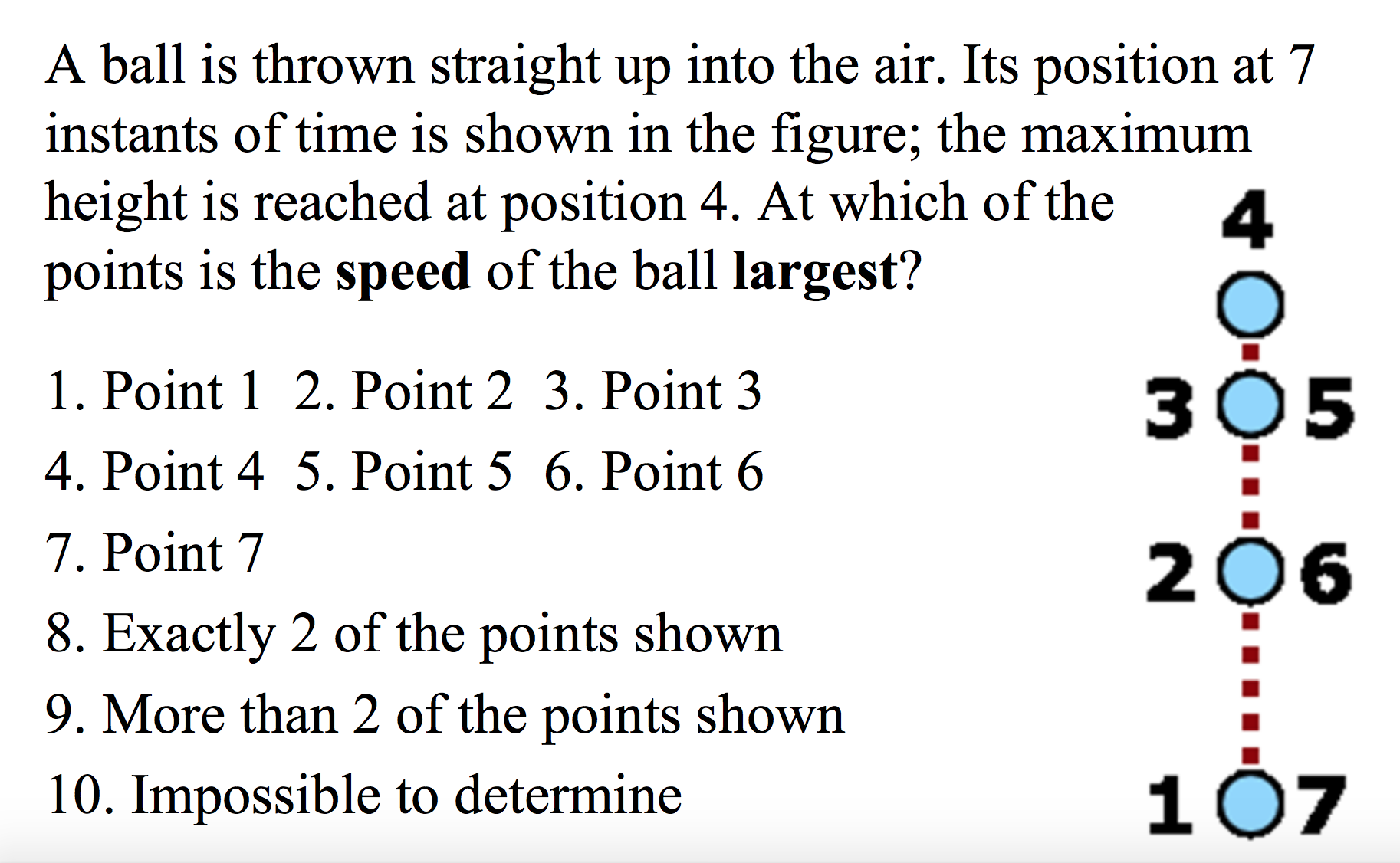# A ball is thrown straight up into the air. Its position at 7instants of time is shown in the figure; the maximumheight is reached at position 4. At which of thepoints is the speed of the ball largest?41. Point 1 2. Point 2 3. Point 33O54. Point 4 5. Point 5 6. Point 67. Point 72068. Exactly 2 of the points shown9. More than 2 of the points shown10710. Impossible to determineO-O.O

Question
41 viewshelp_outlineImage TranscriptioncloseA ball is thrown straight up into the air. Its position at 7 instants of time is shown in the figure; the maximum height is reached at position 4. At which of the points is the speed of the ball largest? 4 1. Point 1 2. Point 2 3. Point 3 3O5 4. Point 4 5. Point 5 6. Point 6 7. Point 7 206 8. Exactly 2 of the points shown 9. More than 2 of the points shown 107 10. Impossible to determine O-O.O fullscreen
check_circle

Step 1

When a ball is thrown up in the air with some velocity, then its velocity decreases with respect to time due to downward force of gravity. After reaching a particular height, the velocity of the ball becomes zero. So, at heighest point of motion, ball is at rest. Just after that the ball starts to fall down freely with an acceleration equal to acceleration due to gravity. The ball starts from zero velocity at the top and due to force of gravity acting in the direction of motion, the velocity of ball increases continuously. Also, during the motion of the ball upto a maximum height and then again back to it’s initial position, the velocity of ball at any two points at the same height is always the same.

Step 2

So, basically the ball’s velocity is maximum at the initial position, and it decreases and becomes zero at the heighest point and then it again increases and becomes equ...

### Want to see the full answer?

See Solution

#### Want to see this answer and more?

Solutions are written by subject experts who are available 24/7. Questions are typically answered within 1 hour.*

See Solution
*Response times may vary by subject and question.
Tagged in

### Kinematics